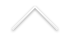Data: 20/08/2019
Horário: 12h
Sala: C116

Palestrante: Reinhard Racke (University of Konstanz, Germany)
Titulo:  The Cauchy Problem for Thermoelastic Plates with Two Temperatures
Resumo:We consider the decay rates of solutions to thermoelastic systems in materials where, in contrast to classical thermoelastic models for Kirchhoff type plates, two temperatures are involved, related by an elliptic equation. The arising initial value problems deal with systems of partial differential equations involving Schr¨odinger like equations, hyperbolic and elliptic equations. Depending on the model – with Fourier or with Cattaneo type heat conduction – we obtain polynomial decay rates without or with regularity loss. This way we obtain another example where the loss of regularity in the Cauchy problem corresponds to the loss of exponential stability in bounded domains. The wellposedness is done using semigroup theory in appropriate space reflecting the different regularity compared to the classical single temperature case, and the (optimal) decay estimates are obtained with sophisticated pointwise estimates in Fourier space.

Palestrante: Ludovick Gagnon (INRIA, França)
Titulo: On the link between controllability and integrability
Resumo: The aim of this talk is to make to present the possible applications of the integrability of a dynamical system (ODE or PDE) to its controllability. On one hand, the integrability, in a broad sense, implies that the dynamical system has more rigidity, either by having more conserved quantities or by having a foliation of its phase space. On the other hand, the controllability refers to the notion of being able to drive the initial state of the dynamical system to another target final state by means of external forces. There exists many methods in the literature to study the controllability of linear ODE or PDE but complications arise quickly when one desires to study the small-time controllability of nonlinear PDEs. To motivate the link between controllability and integrability, we shall first revisit the now well known controllability of the linear wave equation on a smooth bounded domain. We shall prove that the integrability of the ellipse yield a surprising result on the controllable regions for the wave equation. We will then move on to challenging open problems of small-time controllability of some nonlinear PDEs, expose limitations of existing methods and give insights of what integrability may provide for the controllability of these equations.